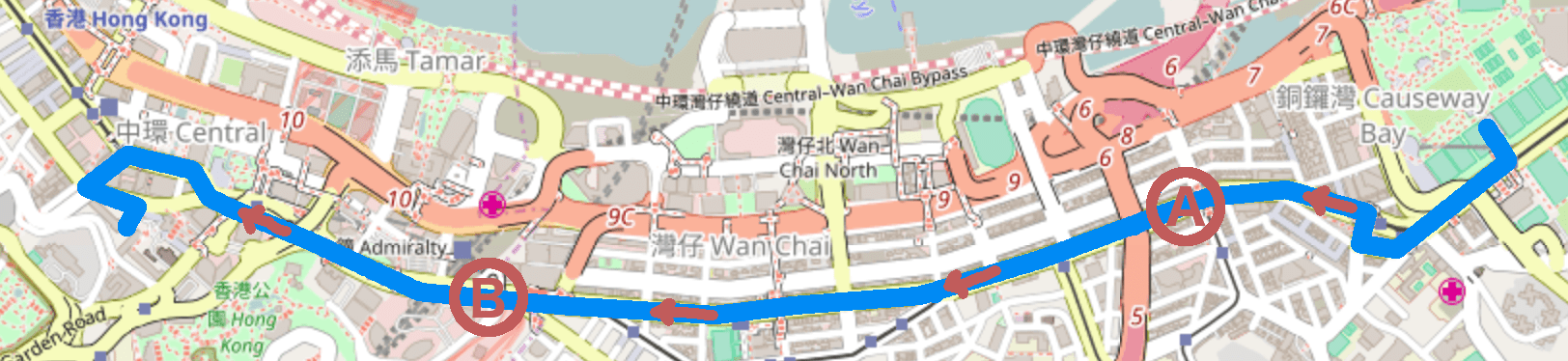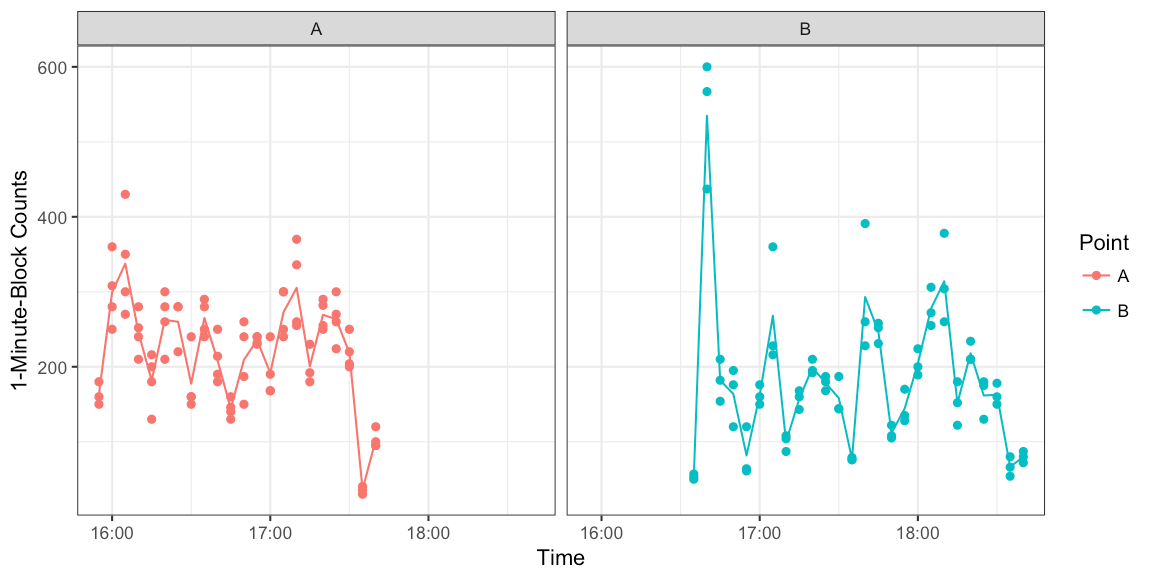Want to share your content on R-bloggers? click here if you have a blog, or here if you don't.

## Abstract

Inspired by the recent March For Science we look into methods for the statistical estimation of the number of people participating in a demonstration organized as a march. In particular, we provide R code to reproduce the two on-the-spot counting method analysis of Yip et al. (2010) for the data of the July 1 March in Hong Kong 2006.## Introduction

Exercising your democratic right to express support for a cause by demonstration has found anew usage. The March for Science is a recent examples of such a demonstration inspired by recent political developments. The number of persons participating in such marches is the indicator by which the support of the cause is measured. Crowd size estimates have therefore always been subject to political interpretation and, hence, possible politically motivated bias. In this work we focus on what statistics has to offer with respect to finding the true number of participants. A good overview of this task’s challenges is given in Watson and Yip (2011). A particular difficulty is the size estimation of moving crowds as seen in marches.

As case study we replicate the analysis of Yip et al. (2010) on estimating the number of participants in the 1st of July Marches in Hong Kong. Since the handover to China in 1997 these marches have been conducted yearly to demonstrate for democracy and freedom of speech in Hong Kong. Below is shown the 3.6 km long demonstration route from Victoria Park to Government Headquarters for the 2006 demonstration as described by Yip et al. (2010). A youtube video of the 2006 demonstration illustrates this better than words.Map Source: Open Street Map

In order to estimate the number of participants a two on-the-spot counting method was devised by Yip et al. (2010): Two points along the march were selected as shown in the above map: Point A denotes the location after which an individual is counted as being part of the march. In order to take into account that people join the march at a later point than A, a second point B is selected to adjust the count at A for such late entries. Three to four persons were stationed at each of the two counting locations. Once the demonstration passed the respective point each of them started to count the number of people passing in a one-minute intervals. They counted for one minute every 5 minutes until the last person of the march had passed the counting point.

We store the resulting counting data displayed in Table 2 and Table 3 of Yip et al. (2010) as two Excel-files. In a data pre-processing step these are then read and combined into one data.frame containing the columns Y1Y4. Furthermore, we re-format the table’s time specification to proper POSIX formatted date-times. The exact data dancing steps can be found in the accompanying Rmd code of this post. Altogether, this yields a tbl with the first couple of lines looking as follows:

## # A tibble: 6 × 7
##      Y1    Y2    Y3    Y4     Mean Point          Time_POSIX
##   <dbl> <dbl> <dbl> <dbl>    <dbl> <chr>              <dttm>
## 1   150    NA   160   180 163.3333     A 2006-07-01 15:55:00
## 2   308   360   250   280 299.5000     A 2006-07-01 16:00:00
## 3   430   350   300   270 337.5000     A 2006-07-01 16:05:00
## 4   210   280   240   252 245.5000     A 2006-07-01 16:10:00
## 5   130   216   200   180 181.5000     A 2006-07-01 16:15:00
## 6   210   260   300   280 262.5000     A 2006-07-01 16:20:00

We then compute a number of row-wise statistics for all columns containing the counts – which columns contain the counts is specified by a regular expression ccol_regexp. In our case would be "^Y[0-9]+".

### Descriptive Statistics

The counts of the 4 counters at point A and the 3 counters at point B are summarized in the following small table:

Point n_counters n_timepoints sum_of_the_mean_counts
A 4 22 4849.50
B 3 26 4746.67

A time series for the individual counts as well as their mean is shown below. One observes that at point B the intensity of the crowd was lower, as the observation had stretched over a larger distance. The later is seen from the time span between the first and last count for the two points: approximately 1:45h for A vs. 2:45h for B.## Two On-the-Spot Counting Method

Below we give the mathematical details of the two on-the-spot counting method. Consider the counting point $$X$$ of the march, i.e. $$X\in \{A,B\}$$. Let $$m_X$$ be the number of counters at this point. Assume that the first people of the march pass $$X$$ at time point $$a_X$$ and that last people of the march reach $$X$$ at time point $$e_X$$. The time unit could for example be minutes. Counting is done such that at regular intervals $$c$$ one counts all people passing the point of observation within a time block of 1 unit – say 1 minute. Let $$k_X$$ denote the number of time points where observations are available at $$X$$. Hence, the $$k_X$$ observations at $$X$$ are available for the time points $$a_X,a_X+c,a_X+2c,a_X+(k_X-1)c$$. Denote by $$Y_{X,j}(t)$$ the $$j$$‘th person’s count at time $$t$$. Then

$\overline{Y}_X(t) = \frac{1}{m_X} \sum_{i=1}^{m_X} Y_{X,i}(t)$

is the average of the observer’s counts at point $$X$$ for time $$t$$. By scaling up each observer’s observations to account for the time blocks without a count and averaging over the different observers we get an estimate for the number of participants at point $$X$$:

$\hat{N}_X = \frac{e_X}{k_X} \sum_{j=1}^{k_X} \overline{Y}_X(a_X + (j-1)c).$

In most cases one would have that $$e_X/k_X=c$$. If a counter thus counts 200 people for every 1-minute-counting-block during two-hours, i.e. corresponding to 24 observations – one every five minutes, her estimate for $$N_X$$ would be 200$$\cdot$$ 24$$\cdot$$ 5= 24000.

In order to adjust the estimate at point $$A$$ for people who joined the march after point $$A$$, we perform an independent counting at point $$B$$ and additionally ask $$m$$ people at point $$A$$, whether they marched past point $$A$$ or not. Denoting $$\hat{\phi}$$ the fraction of people answering yes to this question the two on-the-spot counting estimator is $\hat{N} = \hat{N}_A + (1-\hat{\phi}) \hat{N}_B.$
Note that this estimator does not take into account that people could potentially leave the march between $$A$$ and $$B$$ and that its also possible to join the march after $$B$$. However, the proportion of such participants is assumed to be negligible.

A confidence interval (CI) based on an asymptotic normal assumption can be obtained by deriving that $\operatorname{se}(\hat{N}) = \sqrt{\widehat{\operatorname{Var}}(\hat{N}_A) + (1-\hat{\phi})^2 \widehat{\operatorname{Var}}(\hat{N}_B) + \hat{N}_B^2 \frac{\hat{\phi}(1-\hat{\phi})}{m}},$
where we have used that $\widehat{\operatorname{Var}}(\hat{N}_X) = \frac{e_X^2}{k_X^2} \sum_{j=1}^{k_X} \widehat{\operatorname{Var}}(\overline{Y}_X(a_X + (j-1)c)) = \frac{e_X^2}{k_X^2} \sum_{j=1}^{k_X} \frac{\widehat{\operatorname{Var}}(Y_X(a_X + (j-1)c))}{m_X}$
and $\widehat{\operatorname{Var}}(Y_X(t)) = \frac{1}{m_X-1}\sum_{i=1}^{m_X} (Y_{X,i}(t) – \overline{Y}_X(t)).$

A two-sided $$(1-\alpha)\cdot 100\%$$ CI is then constructed as $$\hat{N} \pm z_{1-\alpha/2} \operatorname{se}(\hat{N})$$. Since $$N$$ is expected to be at least of moderate size before one bothers counting this asymptotic CI should have decent coverage.

### Implementation in R

The above equations have been implemented as function two_on_the_spot_N in R, which given a counts data.frame computes the estimate and an corresponding confidence interval. The github code of this post contains the details.

args(two_on_the_spot_N)

## function (counts, ccol_regexp = "^Y[0-9]+", phi_estim, c = 5,
##     conf.level = 0.95)
## NULL

Among 480 interviewed persons at point B, 437 reported to also have passed point A. In other words $$\hat{\phi}$$=91% and we obtain $$\hat{N}$$ as follows with R:

##Compute the two on the spot estimate based on the data in counts
N <- two_on_the_spot_N(counts, phi_estim=c(437,480),conf.level=0.95)

##Rounded version
with(N, round(c(estimate=estimate,ci)/100)*100)

## estimate ci_lower ci_upper
##    26400    25600    27200

Our estimate for the number of participants is thus around 26400 with a 95% confidence interval of 25600-27200. For comparison the author's state that the Hong Kong Police estimate was around 28000 whereas the organizers claimed a size of 58000.

## Discussion

We were able to reproduce the results of Yip et al. (2010) using the article's data (up to some rounding issues). An R function is now available for supporting mobile crowd estimation in the future. It will be interesting to data synthesize such traditional counting approaches with more modern data sources such as mobile phone or twitter data (Botta, Moat, and Preis 2015).QED.

Botta, Federico, Helen Susannah Moat, and Tobias Preis. 2015. “Quantifying Crowd Size with Mobile Phone and Twitter Data.” Royal Society Open Science 2 (5). The Royal Society. doi:10.1098/rsos.150162.

Watson, Ray, and Paul Yip. 2011. “How Many Were There When It Mattered?” Significance 8 (3): 104–7. doi:10.1111/j.1740-9713.2011.00502.x.

Yip, Paul S. F., Ray Watson, K. S. Chan, Eric H. Y. Lau, Feng Chen, Ying Xu, Liqun Xi, Derek Y. T. Cheung, Brian Y. T. Ip, and Danping Liu. 2010. “Estimation of the Number of People in a Demonstration.” Australian & New Zealand Journal of Statistics 52 (1). Blackwell Publishing Asia: 17–26. doi:10.1111/j.1467-842X.2009.00562.x.